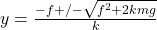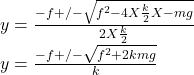## A cylinder of mass mm is free to slide in a vertical tube. The kinetic friction force between the cylinder and the walls of the tube has mag

Question

A cylinder of mass mm is free to slide in a vertical tube. The kinetic friction force between the cylinder and the walls of the tube has magnitude ff. You attach the upper end of a lightweight vertical spring of force constant kk to the cap at the top of the tube, and attach the lower end of the spring to the top of the cylinder. Initially the cylinder is at rest and the spring is relaxed. You then release the cylinder. What vertical distance will the cylinder descend before it comes momentarily to rest? Express your answer in terms of the variables mmm, fff, and constants ggg, kkk.

in progress 0
6 months 2021-08-04T20:04:31+00:00 1 Answers 3 views 0Explanation:

Let y₀ be the initial position of the cylinder when the spring is attached and y its position when it is momentarily at rest.From work-kinetic energy principles,  The work done by the spring force + work done by friction + work done by gravity = kinetic energy change of the cylinder

work done by the spring force = ¹/₂k(y₀² – y²)

work done by friction = – f(y – y₀)

work done by gravity = mg(y – y₀)

kinetic energy change of the cylinder = ¹/₂m(v₁² – v₀²)

So ¹/₂k(y₀² – y²) – f(y – y₀) + mg(y – y₀) = ¹/₂m(v₁² – v₀²)

Since the cylinder starts at rest, v₀ = 0. Also, when it is momentarily at rest, v₁ = 0

¹/₂k(y₀² – y²) – f(y – y₀) + mg(y – y₀) = ¹/₂m(0² – 0²)

¹/₂k(y₀² – y²) – f(y – y₀) + mg(y – y₀) = 0

¹/₂ky₀² + fy₀ – mgy₀ -¹/₂ky² – fy + mgy = 0

¹/₂ky₀² + fy₀ – mgy₀ = ¹/₂ky² + fy – mgy

Let y₀ = 0, then the left hand side of the equation equals zero. So,

0 = ¹/₂ky² + fy – mgy

¹/₂ky² + fy – mgy = 0